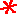Main      Site Guide### Missing Pairs

In each of these puzzles, there are two double blanks. Fill in the blanks with the same pair of letters to complete an English word. For example, use the letter-pair ac in b__ckp__k to make the word backpack.

v _ _ lat _ _ n

Solution

h _ _ dw _ _ e

Solution

_ _ rses _ _ e

Solution

a _ _ a _ _ a

Solution

s _ _ tim _ _ t

Solution

who _ _ sa _ _

Solution

p _ _ sev _ _ e

Solution

## #8tr _ _ bad _ _ r

Solution

_ _ da _ _ ted

Solution

## #10

d _ _ tingu _ _ h

Solution

## #11_ _ s _ _ de

Solution

_ _ od _ _ rk

Solution

p _ _ dl _ _

Solution

_ _ p _ _ zard

Solution

h _ _ g _ _ lin

Solution

c _ _ kb _ _ k

Solution

## #17a _ _ mo _ _

Solution

## #18_ _ at _ _

Solution

s _ _ ur _ _ e

Solution

lo _ _ i _ _

Solution

## #21_ _ i _ _

Solution